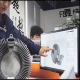# How to Create a Low Leakage Rectifier Using a Self-Powered Op Amp

You can combine a well-chosen op amp, a low-threshold P-channel MOSFET, and two feedback resistors to make a rectifier circuit with a lower forward voltage drop than a diode (Figure 1). The rectified output voltage powers the active circuit, so no additional power supply is required. The quiescent current of this circuit is lower than the reverse leakage current of most Schottky diodes. The circuit provides active rectification when the voltage drops to 0.8V. At lower voltages, the body diode of the MOSFET takes over as a normal diode.

Author: Martin Tomasz

You can combine a well-chosen op amp, a low-threshold P-channel MOSFET, and two feedback resistors to make a rectifier circuit with a lower forward voltage drop than a diode (Figure 1). The rectified output voltage powers the active circuit, so no additional power supply is required. The quiescent current of this circuit is lower than the reverse leakage current of most Schottky diodes. The circuit provides active rectification when the voltage drops to 0.8V. At lower voltages, the body diode of the MOSFET takes over as a normal diode.This circuit simulates a rectifier, but it has a forward voltage drop of 40 mV or less. This circuit has less reverse leakage than a Schottky diode.

When a forward voltage develops between the input and output voltages, the op amp circuit turns on the MOSFET according to the following equation:When a forward voltage develops between the input and output voltages, the op amp circuit turns on the MOSFET according to the following equation:Where VDS is the drain-source voltage and VGS is the gate-source voltage. Combine these equations to relate the gate drive of the MOSFET as a function of drain-source voltage:If you make R2 12 times larger than R1, the 40 mV drop across the MOSFET’s drain-source voltage is sufficient to turn on the MOSFET at low leakage currents (Figure 5). You can choose a higher ratio to further reduce the dropout within the op amp’s worst-case input offset limit of 6 mV. The op amp is powered by the output storage capacitor C1. The amplifier has rail-to-rail inputs and outputs and no phase inversion when operating close to rail. The amplifier operates from supply voltages as low as 0.8V. You connect the non-inverting input of the op amp directly to the VDD rail and the output of the amplifier to the gate of the MOSFET. This circuit consumes slightly more than 1µA when actively rectifying a 100Hz sine wave, which is less leakage current than most Schottky diodes.The output of a circuit (green) with a sine wave input (yellow) shows that the gate voltage of the FET (blue) only drops when the input-to-output differential is less than 40 mV.

The bandwidth of the op amp limits the circuit to low frequency signals. At bandwidths above 500 Hz, the amplifier’s gain begins to drop. As the signal frequency increases, the MOSFET remains off and the body diode of the MOSFET takes over the rectification function. An input with a fast fall time may drag the output with reverse current through the MOSFET. However, for small currents, the MOSFET operates within its subthreshold range. Due to the exponential relationship between gate-to-source voltage and drain-to-source current in the subthreshold range, the amplifier turns off rapidly. The limiting factor is the amplifier’s 1.5V/msec slew rate. As long as your circuit load does not drive the MOSFET into its linear range, the reverse current will not exceed the forward current.

You can use this circuit in micropower solar harvesting applications (Figure 6). Depending on the light, the BPW34 cell produces 10 to 30 μA at voltages of 0.8 to 1.5V. The active diode circuit rectifies the peak harvesting voltage under rapidly changing light conditions and minimizes reverse leakage to the battery.You can use an active rectifier circuit to charge the capacitor of the solar cell. The rectifier has a low voltage drop to protect the battery from reverse current when there is no light.Author: Yoyokuo